Math resources Geometry

3D shapes

Triangular prism

# Triangular prism

Here you will learn about triangular prisms, including how to classify and identify triangular prisms.

Students will first learn about triangular prisms as a part of geometry in 1 st grade and will continue to work with them into middle school.

## What are triangular prisms?

A triangular prism is a polyhedron (3D shape made from polygons) consisting of two triangular ends connected by three rectangles. The triangular ends of a triangular prism are congruent (exactly the same).

For example,

## Properties of a triangular prism

The properties of a triangular prism include faces, edges and vertices (corners).

Faces

A face of a 3D shape is a flat surface.

Triangular prisms have a total of \textbf{5} faces – 2 triangular faces and 3 rectangular faces.

The triangular faces of a triangular prism are congruent.

Edges

An edge of a 3D shape is a straight line between two faces.

Triangular prisms have \textbf{9} edges:

Vertices

A vertex is a point where two or more edges meet.

Triangular prisms have \textbf{6} vertices:

## Labeling a triangular prism

You can label the vertices (corners) of a prism to help identify certain edges or faces.

For example,

Using this labeling, you can identify lengths.

For example, the length AB :

You can also identify faces,

For example, the face ABC :

You can also calculate the volume and surface area of triangular prisms. These are topics that will be covered in middle school.

## Volume of a triangular prism

The volume of a triangular prism is how much space there is inside of the shape.

To calculate the volume of a triangular prism, find the area of the triangular cross-section and multiply it by the length of the prism (or height of the prism).

\text {Volume of a triangular prism }=\text {Area of triangular cross section } \times \text { length }

For example, find the volume of the right triangular prism (so called because the triangular faces include a right angle).

\begin{aligned} & \text{Area of triangular base:}\\\\ & \text {Area }=\cfrac{1}{2} \, bh\\\\ & \text {Area }=\cfrac{1}{2} \times 2 \times 4\\\\ & \text {Area }=4 \mathrm{~cm}^{2}\\ \end{aligned}

\begin{aligned} & \text{Volume of triangular prism:}\\\\ & \text{Volume }= \text{Area of triangular base } \times \text{ length}\\\\ & \text{Volume }=4 \times 7\\\\ & \text{Volume }=28\mathrm{~cm}^{3} \end{aligned}

Volume is measured in cubic units (for example, \mathrm{mm}^3, \mathrm{cm}^3, \mathrm{m}^3, \, etc).

## Surface area of a triangular prism

The surface area of a triangular prism is the total area of all of the faces.

To calculate the surface area of a triangular prism, find the area of each face and add them all together.

Lateral faces are all of the faces of an object excluding the top and the base. For a triangular prism the top and the base are triangles and the lateral faces are rectangular sides. The lateral surface area is the total area of the rectangular sides.

The triangular faces of a triangular prism are congruent (exactly the same) but, unless the triangle is an isosceles triangle or an equilateral triangle, the rectangles are all different.

For example,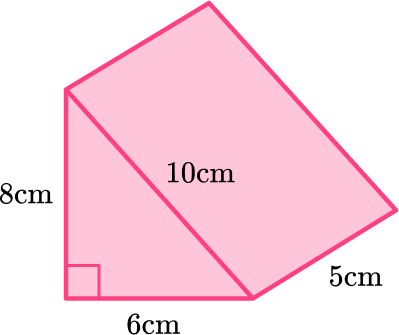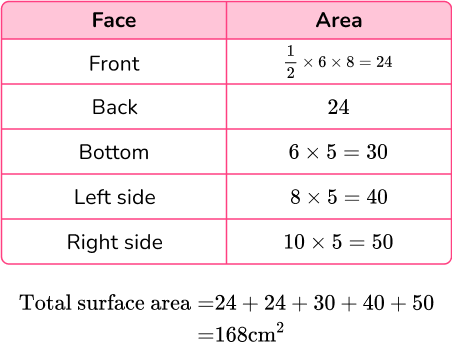Since it is an area, surface area is measured in square units (for example, \mathrm{mm}^2, \mathrm{cm}^2, \mathrm{m}^2, \, etc).

### What are triangular prisms?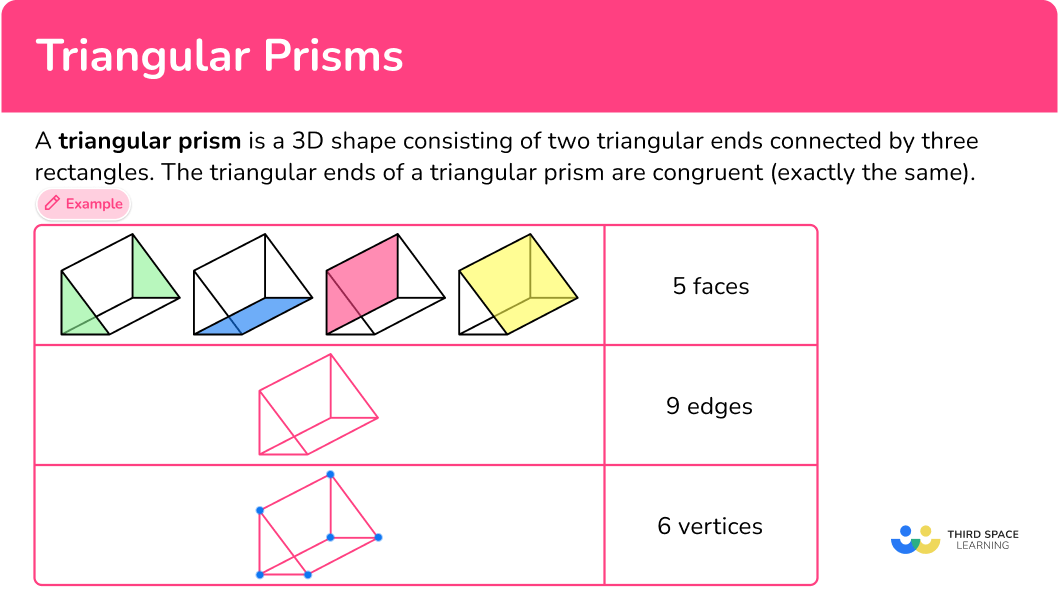## Common Core State Standards

How does this relate to 1 st and 7 th grade math?

Compose two-dimensional shapes (rectangles, squares, trapezoids, triangles, half-circles, and quarter-circles) or three-dimensional shapes (cubes, right rectangular prisms, right circular cones, and right circular cylinders) to create a composite shape, and compose new shapes from the composite shape.

Solve real-world and mathematical problems involving area, volume and surface area of two- and three-dimensional objects composed of triangles, quadrilaterals, polygons, cubes, and right prisms.

## How to identify a triangular prism

In order to identify a triangular prism, you will:

1. Look for the characteristics of a triangular prism.
2. State whether or not the shape is a triangular prism.
3. If the shape is not a triangular prism, explain what characteristics are different.

## Triangular prisms examples

### Example 1: triangular prisms

Look at the shape below and determine if it is a triangular prism or not.

1. Look for the characteristics of a triangular prism.

A triangular prism has 2 triangular faces on the ends that are connected by three rectangles faces.

This shape has a square base with 4 triangular sides.

2State whether or not the shape is a triangular prism.

This shape is a pyramid, not a triangular prism.

3If the shape is not a triangular prism, explain what characteristics are different.

The shape has a square base with 4 triangular sides.

### Example 2: triangular prisms

Look at the shape below and determine if it is a triangular prism or not.

A triangular prism has 2 triangular faces on the ends that are connected by three rectangles faces.

This shape has 2 triangular faces,

and 3 rectangular faces.

This shape is a triangular prism because it has 2 triangular faces and 3 rectangular faces.

### Example 3: triangular prisms

Look at the shape below and determine if it is a triangular prism or not.

A triangular prism has 2 triangular faces on the ends that are connected by three rectangles faces.

This shape has 6 rectangular faces.

This shape is not a triangular prism, it is a rectangular prism.

A rectangular prism has 6 rectangular faces.

### Example 4: triangular prisms (real-life example)

Look at the shape below and determine if it is a triangular prism or not.

A triangular prism has 2 triangular faces on the ends that are connected by three rectangles faces.

This shape has 2 triangular faces.

And 3 rectangular faces.

This shape is a triangular prism.

## How to calculate the volume of a triangular prism

In order to calculate the volume of a triangular prism:

1. Write down the formula.
2. Calculate the area of the base.
3. Calculate the volume of the triangular prism.
4. Write the answer, including the units.

### Example 5: volume of a triangular prism (7th grade question)

Calculate the volume of the triangular prism.

\text {Volume }=\text {Area of triangular base } \times \text { length }

The base of the triangle is 10{~cm} .

The height of the triangle is 4{~cm} .

The area formula for a triangle is:

\begin{aligned} & \text{Area of triangle }=\cfrac{1}{2} \times b \times h\\\\ & \text{Area of triangle }=\cfrac{1}{2} \times 10 \times 4\\\\ & \text{Area of triangle }=20 \end{aligned}

The volume formula is:

\begin{aligned} & \text {Volume of triangular prism }=\text {Area of triangular base } \times \text { length }\\\\ & \text{Volume of triangular prism }=20 \times 15\\\\ & \text{Volume of triangular prism }=300 \end{aligned}

The measurements on this triangular prism are in cm so the volume will be measured in {cm}^3 .

\text {Volume }=300 \mathrm{~cm}^3

## How to calculate the surface area of a triangular prism

In order to calculate the surface area of a triangular prism:

1. Calculate the area of each face.
2. Add the area of each face together.
3. Include the units.

### Example 6: surface area of a triangular prism (7th grade question)

Calculate the surface area of the triangular prism.

\text {Total surface area: } 24 + 24 + 24 + 32 + 40 = 144

The measurements on the triangular prism are in mm therefore the total surface area of the triangular prism = 144 \mathrm{~mm}^2 .

### Teaching tips for triangular prism

• Start by showing a physical triangular prism to the students. You can use a transparent plastic model or create one using cardboard. This hands-on approach helps students visualize the shape and understand its properties.

• Connect the concept of triangular prisms to objects that students encounter in their daily lives. For example, you can mention buildings with triangular roofs or packaged items with triangular prism shapes.

• Instead of practicing with worksheets, use activities that involve constructing or creating triangular prisms. Provide materials like straws, clay, or blocks that students can use to build their own models of triangular prisms.

### Easy mistakes to make

• Confusing triangular prism with other shapes
Students might identify other geometric shapes as triangular prisms. For example, students might confuse it with a pyramid or a cone due to the presence of a triangular base.

• Incorrectly identifying the edges and vertices
Students might struggle with counting the edges and vertices of a triangular prism accurately. They may overloop some edges, or mistakenly count the same edge or vertex twice.

• Using the wrong measurements to work out the area of the triangle faces
In surface area questions, you need to know all three side lengths of the triangle. However you only need the base and the height to calculate the area of the triangle.

### Practice triangular prism questions

1) Which shape is a triangular prism?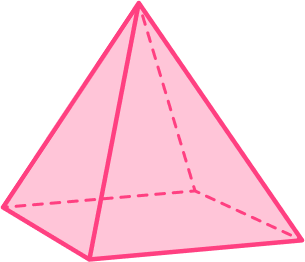This shape has two triangular bases and three rectangular faces.

This shape is a triangular prism.2) Which shape is a triangular prism?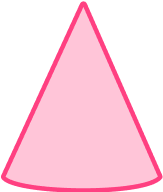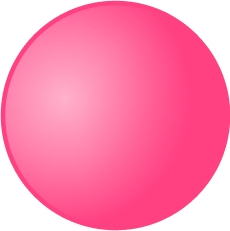This shape has two triangular bases and three rectangular faces.

This shape is a triangular prism.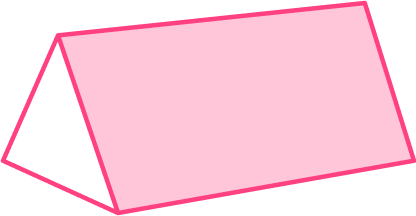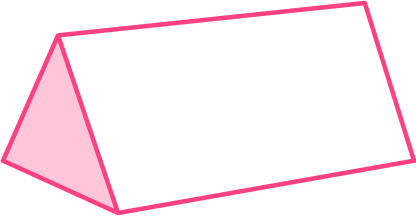3) Which shape is a triangular prism?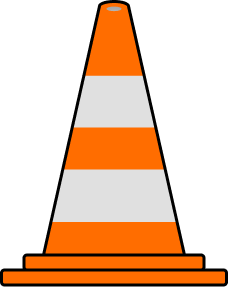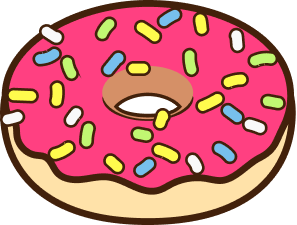This shape has two triangular bases and three rectangular faces.

This shape is a triangular prism.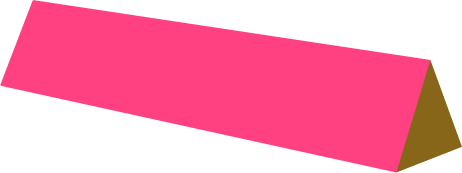4) Which shape is NOT a triangular prism?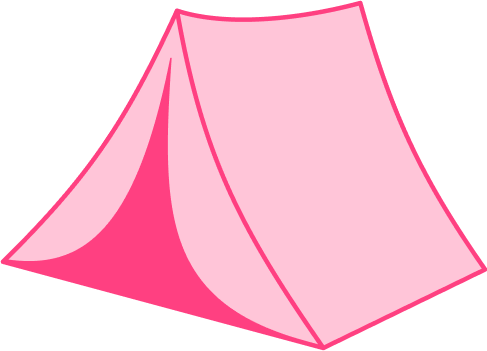This shape has one base, but it does not have any rectangular or triangular faces. This shape is a cone.

5) The volume of this prism is 96{~cm}^2 . Find the height, h , of the prism.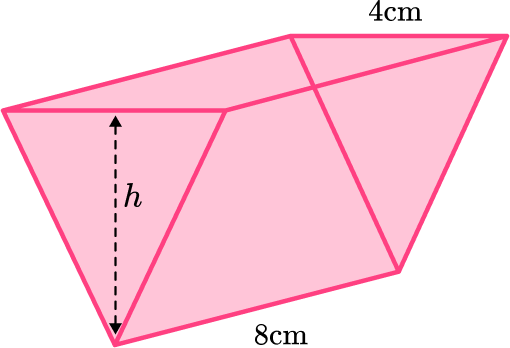32{~cm}16{~cm}3{~cm}6{~cm}\begin{aligned} \text{Area of triangle }&=\cfrac{1}{2} \times 4 \times h\\\\ &=2h \end{aligned}

\begin{aligned} \text{Volume of triangular prism }&= 2h \times 8\\\\ 96 &= 16h\\\\ 6&=h \end{aligned}

The height is 6{~cm} .

6) Calculate the surface area of the triangular prism.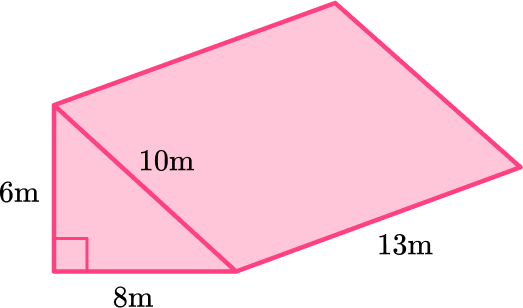312 \mathrm{~m}^{2}438 \mathrm{~m}^{2}360 \mathrm{~m}^{2}408 \mathrm{~m}^{2}Work out the area of each face: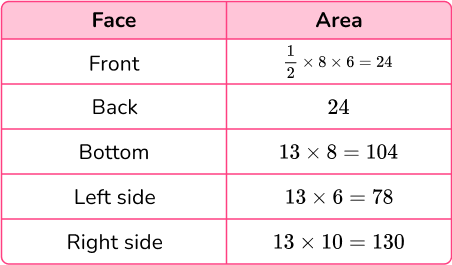\text{Total surface area }=24+24+104+78+130=360\mathrm{~m}^{2}

## Triangular prism FAQs

Can a triangular prism have equal sides or angles?

Yes, a triangular prism can have equal sides and angles. If the triangular base is an equilateral triangle (all sides and angles are equal), and the height of the prism is perpendicular to the base, then all sides and angles of the prism will be equal.

What is the difference between a triangular prism and a rectangular prism?

The main difference between a rectangular prism and a triangular prism is that a rectangular prism has rectangular bases and rectangular sides, while a triangular prism has triangular bases and rectangular faces. The number of edges and vertices also differs between the two shapes.

What are examples of other types of prisms?

Regular triangular prisms are not the only type of prism you will learn about in your math career. As you progress through math, you will learn about other prisms including: hexagonal prisms, right triangular prisms, and oblique triangular prisms to name a few.

Can you use the pythagorean theorem to triangular prisms?

To apply the Pythagorean theorem in the context of a triangular prism, you can use it to find the lengths of the sides of the triangular base if you know the lengths of the other sides. Then, you can use those lengths to calculate the area of base, which is needed for finding the surface area or volume of the prism.

## Still stuck?

At Third Space Learning, we specialize in helping teachers and school leaders to provide personalized math support for more of their students through high-quality, online one-on-one math tutoring delivered by subject experts.

Each week, our tutors support thousands of students who are at risk of not meeting their grade-level expectations, and help accelerate their progress and boost their confidence.